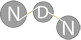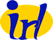NS-3 based Named Data Networking (NDN) simulator ndnSIM 2.0: NDN, CCN, CCNx, content centric networks API Documentation
nfd::RttEstimator Class Reference

implements the Mean-Deviation RTT estimator More...

`#include <rtt-estimator.hpp>`

## Public Types

typedef time::microseconds Duration

## Public Member Functions

RttEstimator (uint16_t maxMultiplier=16, Duration minRto=time::milliseconds(1), double gain=0.1)

void incrementMultiplier ()

void doubleMultiplier ()

Duration computeRto () const

## Static Public Member Functions

static Duration getInitialRtt (void)

## Detailed Description

implements the Mean-Deviation RTT estimator

reference: ns3::RttMeanDeviation

This RttEstimator algorithm is designed for TCP, which is a continuous stream. NDN Interest-Data traffic is not always a continuous stream, so NDN may need a different RttEstimator. The design of a more suitable RttEstimator is a research question.

Definition at line 43 of file rtt-estimator.hpp.

## Member Typedef Documentation

 typedef time::microseconds nfd::RttEstimator::Duration

Definition at line 46 of file rtt-estimator.hpp.

## Constructor & Destructor Documentation

 nfd::RttEstimator::RttEstimator ( uint16_t maxMultiplier = `16`, Duration minRto = `time::milliseconds(1)`, double gain = `0.1` )

Definition at line 30 of file rtt-estimator.cpp.

Referenced by getInitialRtt().

## Member Function Documentation

 static Duration nfd::RttEstimator::getInitialRtt ( void )
inlinestatic

Definition at line 49 of file rtt-estimator.hpp.

 void nfd::RttEstimator::addMeasurement ( Duration measure )

Definition at line 42 of file rtt-estimator.cpp.

Referenced by getInitialRtt().

 void nfd::RttEstimator::incrementMultiplier ( )

Definition at line 60 of file rtt-estimator.cpp.

Referenced by getInitialRtt().

 void nfd::RttEstimator::doubleMultiplier ( )

Definition at line 66 of file rtt-estimator.cpp.

Referenced by getInitialRtt().

 RttEstimator::Duration nfd::RttEstimator::computeRto ( ) const

Definition at line 72 of file rtt-estimator.cpp.

Referenced by getInitialRtt().

The documentation for this class was generated from the following files: